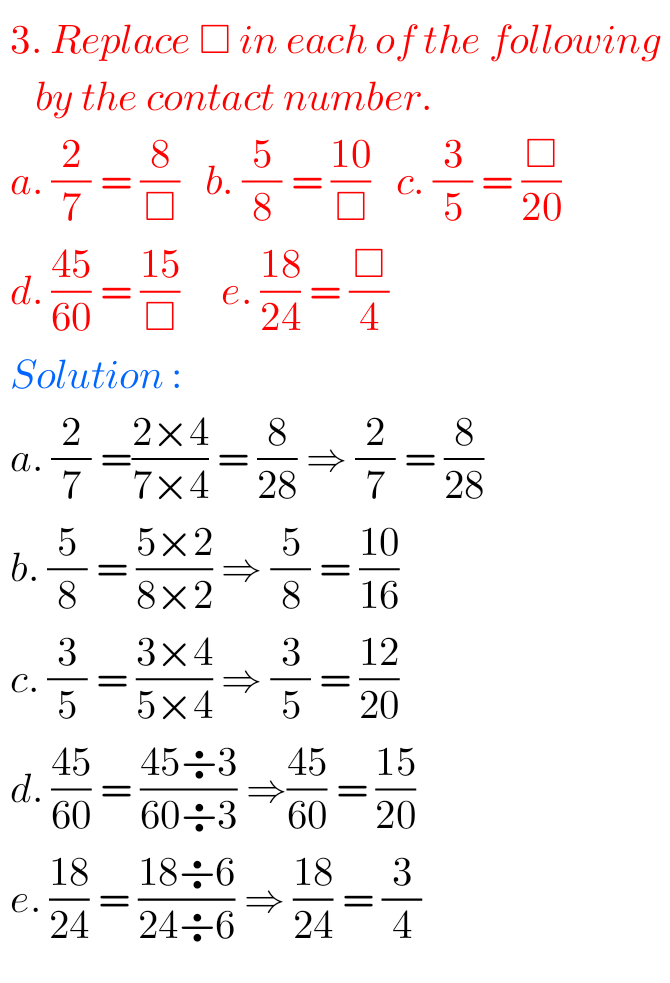## Maths Solutions for Ncert Fractions exercise 7.3

Ncert solutions for Fractions exercise 7.3 class 6 Maths Ncert Ncert Maths Class 6 Chapter 7 exercise 7.3 textbook problems with solutions are given below. Study the textbook lesson Fractions very well. Observe the example problems and solutions given in the textbook. Try them. Observe the given below solutions and try them in your own …